### Magnetic dipole:

An arrangement of two equal and opposite magnetic poles separated by a small distance is called a magnetic dipole.

### Magnetic dipole moment:

The magnetic dipole moment of a magnetic dipole is defined as the product of its pole strength and magnetic length. It is a vector quantity, directed from S-pole to N-pole.

Ampere found that the distribution of magnetic lines of force around a finite current carrying solenoid is similar to that produced by a bar magnet.

The magnetic induction at a point along the axis of a circular coil carrying current is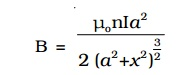The direction of this magnetic field is along the axis and is given by right hand rule. For points which are far away from the centre of the coil, x>>a, a2 is small and it is neglected. Hence for such points,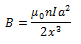If we consider a circular loop, n = 1, its area A = πa2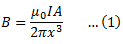The magnetic induction at a point along the axial line of a short bar magnet is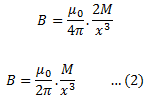Comparing equations (1) and (2), we find that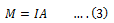Hence a current loop is equivalent to a magnetic dipole of moment M = IA

The magnetic moment of a current loop is defined as the product of the current and the loop area. Its direction is perpendicular to the plane of the loop.